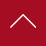• 保险
• 年终奖
• 工作餐
• 公费旅游
• 公费培训
• 双休

• 保险
• 年终奖
• 公费旅游
• 公费培训
• 标准工作时间
• 带薪休假

• 带薪年假
• 五险
• 团队氛围好
• 工作氛围好
• 晋升空间大

• 保险
• 工作餐
• 公费培训
• 双休
• 双休
• 标准工作时间

• 保险
• 年终奖
• 工作餐
• 公费旅游
• 公费培训
• 标准工作时间

• 全勤奖
• 优秀员工奖
• 团队氛围好
• 工作氛围好
• 晋升空间大

• 五险一金
• 用餐补贴
• 年终奖金
• 带薪休假

• 年终奖
• 工作餐
• 公费旅游
• 公费培训
• 双休

• 年终奖
• 公费旅游
• 公费培训
• 标准工作时间
• 带薪休

• 全勤奖
• 五险
• 住房补贴
• 晋升空间大
• 工作氛围好
• 团队氛围好

• 底薪+餐补+提成+奖金+日常福利+年终奖+月休4天

• 底薪+餐补+住房+提成+奖金+日常福利+月休4天

• 保险
• 年终奖
• 公费旅游
• 公费培训
• 标准工作时间
• 带薪休假

• 公费培训
• 标准工作时间
• 带薪休
• 年轻化
• 团队氛围好
• 晋升空间大

• 1
• 2
•••••